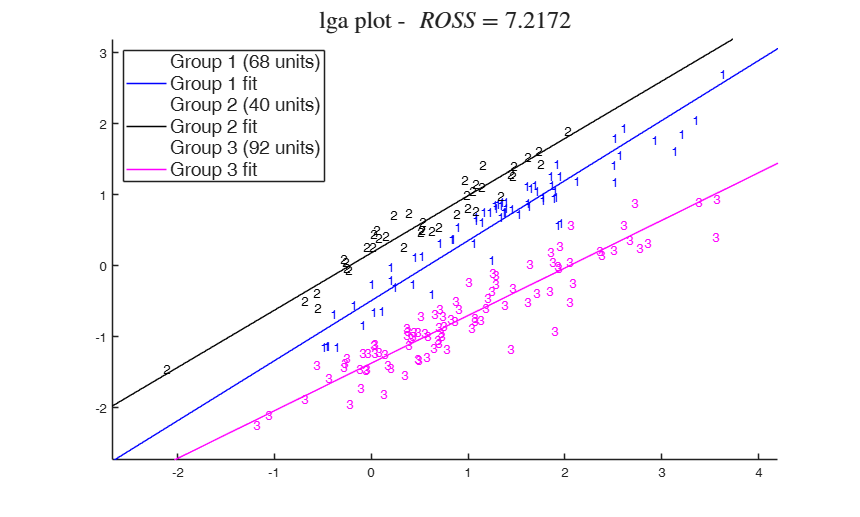# lga

lga performs linear grouping analysis

## Syntax

• out=lga(X,k)example
• out=lga(X,k,Name,Value)example

## Description

 out =lga(X, k) lga with all default options.

 out =lga(X, k, Name, Value) lga with niter = 1000 and biter = 3000.

## Examples

expand all

###lga with all default options.

rng('default')
rng(123); % this leads to ROSS = 7.2172;
out=lga(X,3);
LGA Algorithm k =3 biter =23 niter =10
Finished.### lga with niter = 1000 and biter = 3000.

X=load('X.txt');
out=lga(X,4,'niter',1000,'biter',3000);

## Input Arguments

### X — input data matrix. Matrix.

Input data as matrix of size n-by-p

Data Types: single| double

### k — number of clusters. Scalar.

Scalar which specifies the number of clusters.

Data Types: single| double

### Name-Value Pair Arguments

Specify optional comma-separated pairs of Name,Value arguments. Name is the argument name and Value is the corresponding value. Name must appear inside single quotes (' '). You can specify several name and value pair arguments in any order as  Name1,Value1,...,NameN,ValueN.

Example:  'biter',1 , 'niter',1 , 'showall','true' , 'stand','true' , 'silent','true' , 'plots',1 

### biter —Hyperplane number.integer.

number of different starting hyperplanes to try.

Example:  'biter',1 

Data Types: double

### niter —Number of iterations.positive integer.

Number of iterations to attempt for convergence.

Example:  'niter',1 

Data Types: double

### showall —Type of display.logical.

If true then display all the outcomes, not just the best one.

Example:  'showall','true' 

Data Types: char

### stand —Data standardization.logical.

If true standardize the X matrix with the standard deviation before fitting.

Example:  'stand','true' 

Data Types: char

### silent —Text ouptut.logical.

If true, produces no text output during processing. The default value is false.

Example:  'silent','true' 

Data Types: char

### plots —plot on the screen.scalar.

If plots=1 a plot is showed on the screen with the final allocation (and if size(X,2)==2 with the lines associated to the groups).

Example:  'plots',1 

Data Types: double

## Output Arguments

### out — description Structure

Structure which contains the following fields

Value Description
cluster

vector containing the cluster memberships.

ROSS

the Residual Orthogonal Sum of Squares for the solution.

converged

logical. True if at least one solution has converged.

nconverg

the number of converged solutions (out of biter starts).

X

the (scaled if selected) dataset.

hpcoeff

best hyerplane

scaled

logical. Is the data scaled?

k

the number of clusters to be found.

biter

the biter setting used.

niter

the niter setting used.

alpha

level of trimming. Added for consistency with rlga. In this case out.alpha=0;

class

'lga'.

Van Aelst, S. and Wang, X. and Zamar, R. and Zhu, R. (2006), Linear Grouping Using Orthogonal Regression, "Computational Statistics and Data Analysis", Vol. 50, pp. 1287-1312.